# 1 Linear Equations in Linear Algebra 1 3

• Slides: 181 Linear Equations in Linear Algebra 1. 3 VECTOR EQUATIONS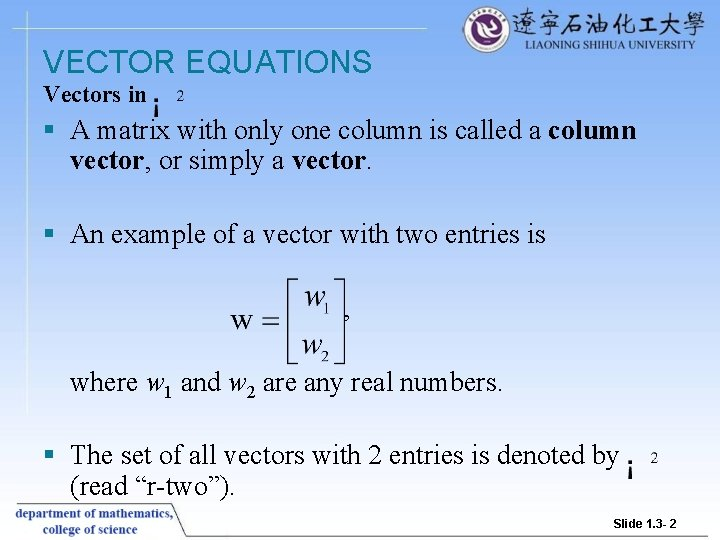VECTOR EQUATIONS Vectors in § A matrix with only one column is called a column vector, or simply a vector. § An example of a vector with two entries is , where w 1 and w 2 are any real numbers. § The set of all vectors with 2 entries is denoted by (read “r-two”). Slide 1. 3 - 2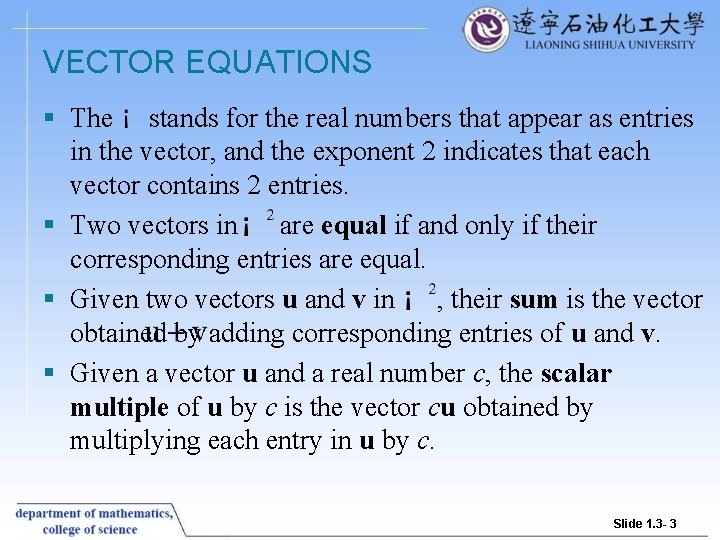VECTOR EQUATIONS § The stands for the real numbers that appear as entries in the vector, and the exponent 2 indicates that each vector contains 2 entries. § Two vectors in are equal if and only if their corresponding entries are equal. § Given two vectors u and v in , their sum is the vector obtained by adding corresponding entries of u and v. § Given a vector u and a real number c, the scalar multiple of u by c is the vector cu obtained by multiplying each entry in u by c. Slide 1. 3 - 3VECTOR EQUATIONS § Example 1: Given 4 u, Solution: and , find . , and Slide 1. 3 - 4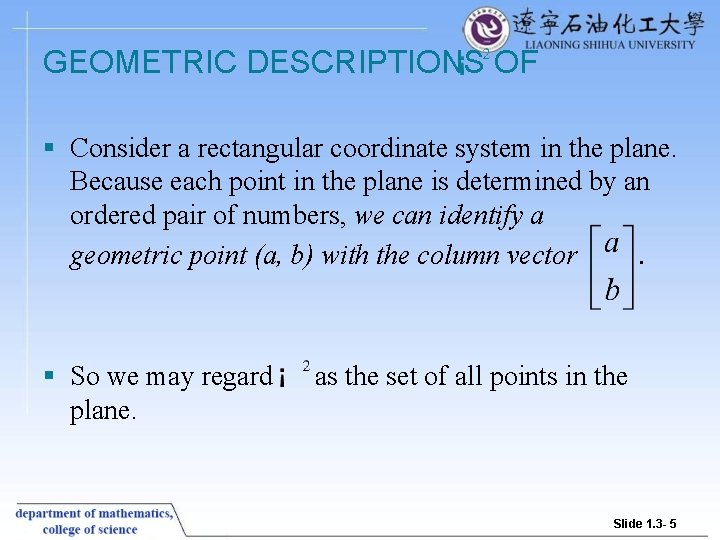GEOMETRIC DESCRIPTIONS OF § Consider a rectangular coordinate system in the plane. Because each point in the plane is determined by an ordered pair of numbers, we can identify a geometric point (a, b) with the column vector. § So we may regard plane. as the set of all points in the Slide 1. 3 - 5PARALLELOGRAM RULE FOR ADDITION § If u and v in are represented as points in the plane, then corresponds to the fourth vertex of the parallelogram whose other vertices are u, 0, and v. See the figure below. Slide 1. 3 - 6VECTORS IN and § Vectors in are column matrices with three entries. § They are represented geometrically by points in a three-dimensional coordinate space, with arrows from the origin. § If n is a positive integer, (read “r-n”) denotes the collection of all lists (or ordered n-tuples) of n real numbers, usually written as column matrices, such as . Slide 1. 3 - 7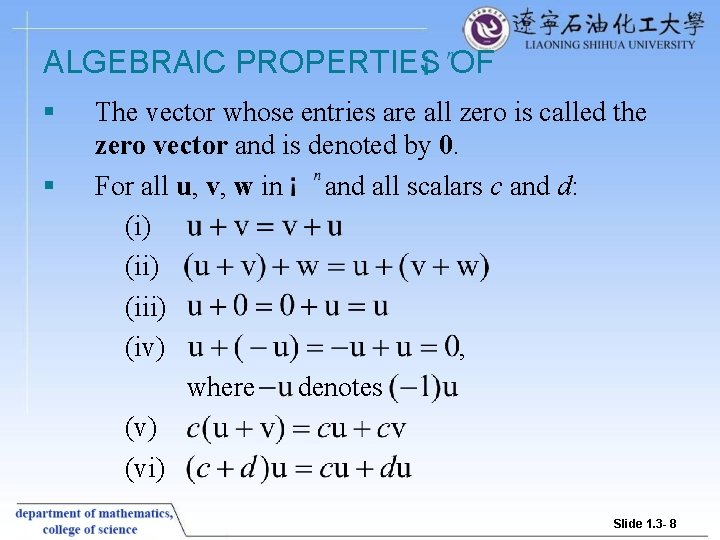ALGEBRAIC PROPERTIES OF § § The vector whose entries are all zero is called the zero vector and is denoted by 0. For all u, v, w in and all scalars c and d: (i) (iii) (iv) , where denotes (v) (vi) Slide 1. 3 - 8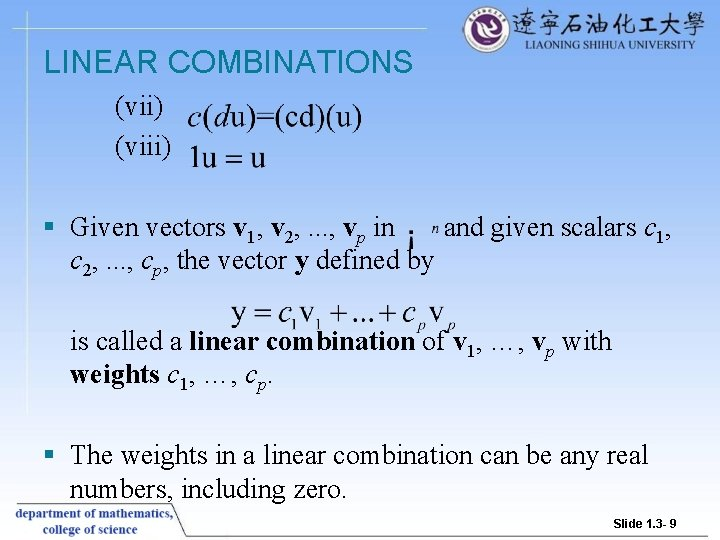LINEAR COMBINATIONS (vii) (viii) § Given vectors v 1, v 2, . . . , vp in and given scalars c 1, c 2, . . . , cp, the vector y defined by is called a linear combination of v 1, …, vp with weights c 1, …, cp. § The weights in a linear combination can be any real numbers, including zero. Slide 1. 3 - 9LINEAR COMBINATIONS § Example 2: Let , and . Determine whether b can be generated (or written) as a linear combination of a 1 and a 2. That is, determine whether weights x 1 and x 2 exist such that ----(1) If vector equation (1) has a solution, find it. Slide 1. 3 - 10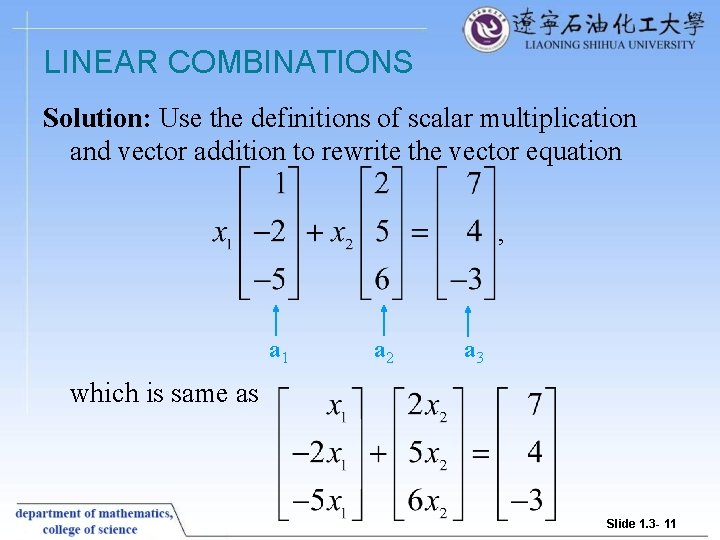LINEAR COMBINATIONS Solution: Use the definitions of scalar multiplication and vector addition to rewrite the vector equation , a 1 a 2 a 3 which is same as Slide 1. 3 - 11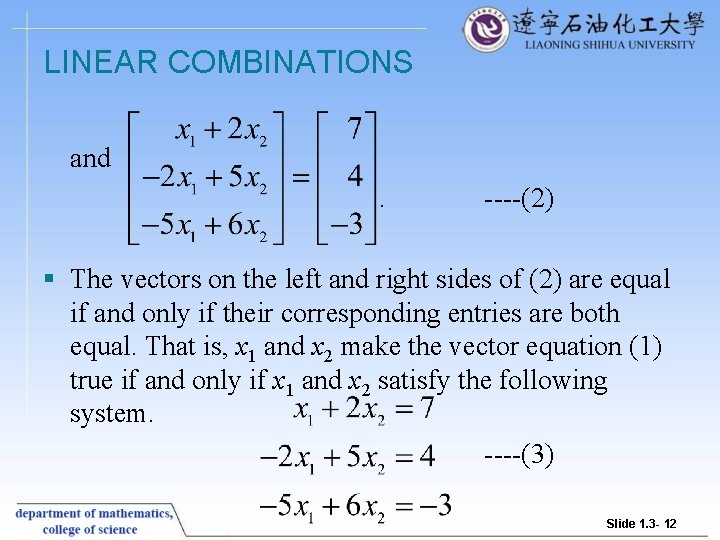LINEAR COMBINATIONS and. ----(2) § The vectors on the left and right sides of (2) are equal if and only if their corresponding entries are both equal. That is, x 1 and x 2 make the vector equation (1) true if and only if x 1 and x 2 satisfy the following system. ----(3) Slide 1. 3 - 12LINEAR COMBINATIONS § To solve this system, row reduce the augmented matrix of the system as follows. § The solution of (3) is and. Hence b is a linear combination of a 1 and a 2, with weights and. That is, . Slide 1. 3 - 13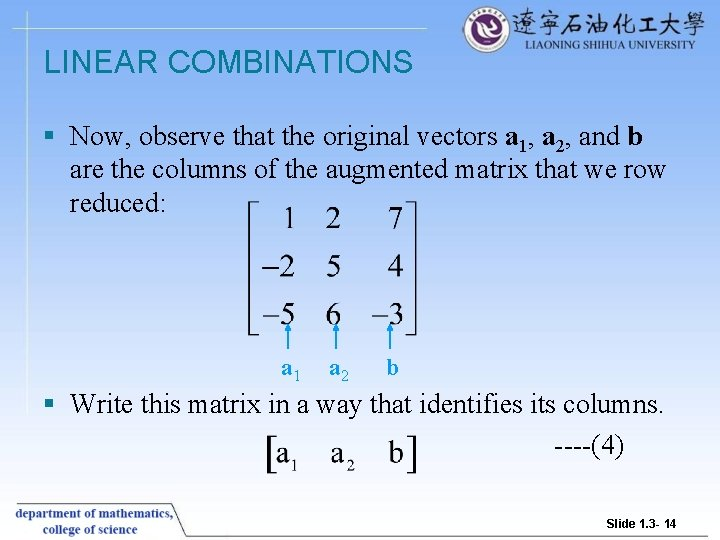LINEAR COMBINATIONS § Now, observe that the original vectors a 1, a 2, and b are the columns of the augmented matrix that we row reduced: a 1 a 2 b § Write this matrix in a way that identifies its columns. ----(4) Slide 1. 3 - 14LINEAR COMBINATIONS § A vector equation has the same solution set as the linear system whose augmented matrix is. ----(5) § In particular, b can be generated by a linear combination of a 1, …, an if and only if there exists a solution to the linear system corresponding to the matrix (5). Slide 1. 3 - 15LINEAR COMBINATIONS § Definition: If v 1, …, vp are in , then the set of all linear combinations of v 1, …, vp is denoted by Span {v 1, …, vp} and is called the subset of spanned (or generated) by v 1, …, vp. That is, Span {v 1, . . . , vp} is the collection of all vectors that can be written in the form with c 1, …, cp scalars. Slide 1. 3 - 16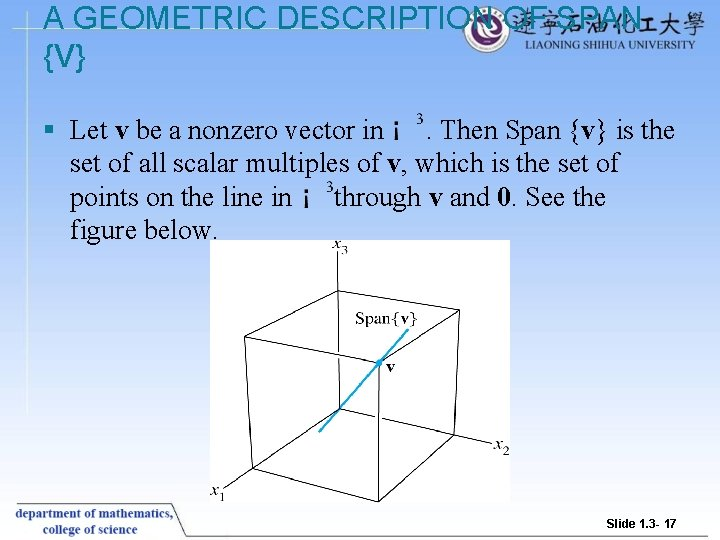A GEOMETRIC DESCRIPTION OF SPAN {V} § Let v be a nonzero vector in. Then Span {v} is the set of all scalar multiples of v, which is the set of points on the line in through v and 0. See the figure below. Slide 1. 3 - 17A GEOMETRIC DESCRIPTION OF SPAN {U, V} § If u and v are nonzero vectors in , with v not a multiple of u, then Span {u, v} is the plane in that contains u, v, and 0. § In particular, Span {u, v} contains the line in through u and 0 and the line through v and 0. See the figure below. Slide 1. 3 - 18